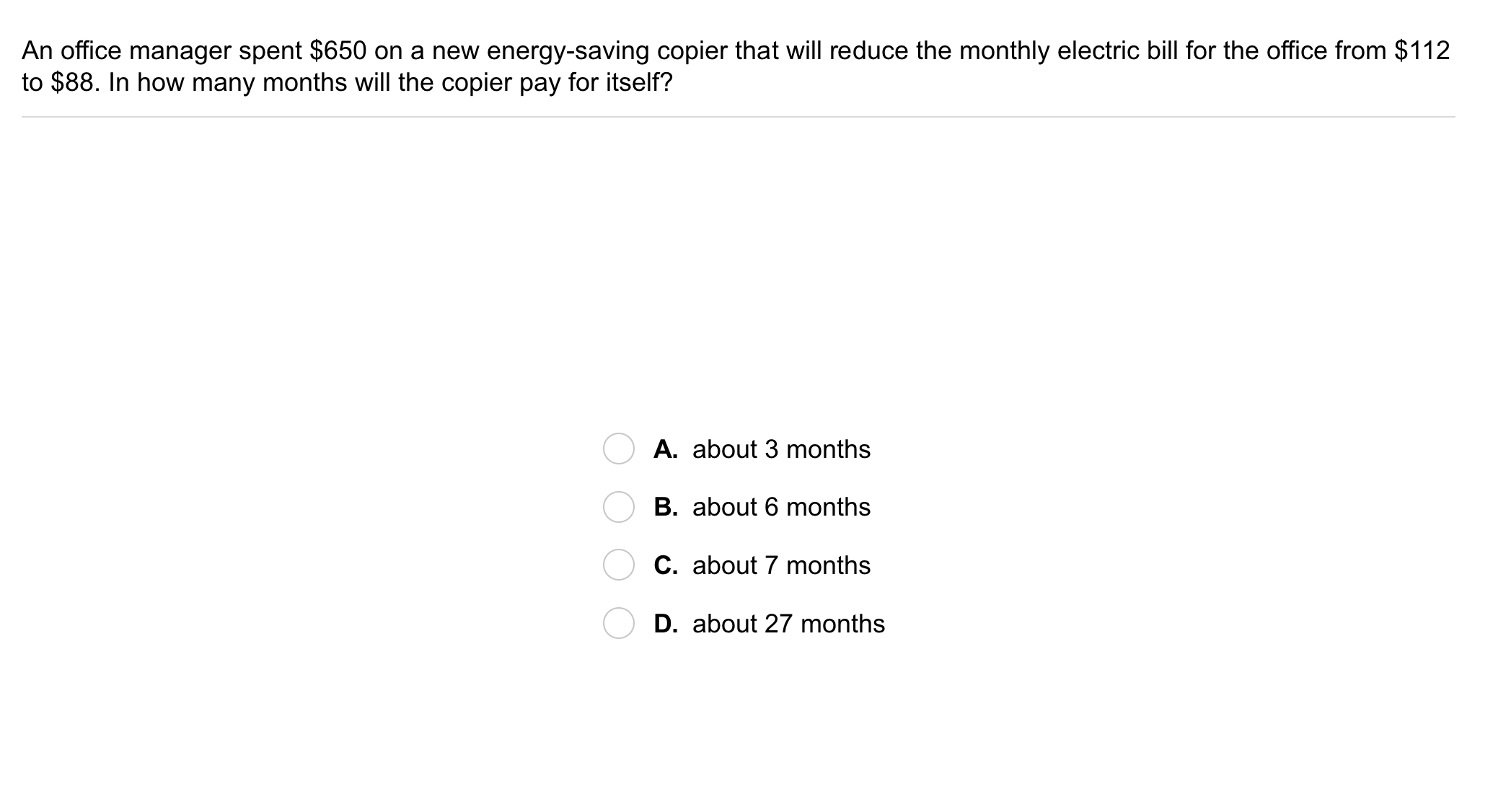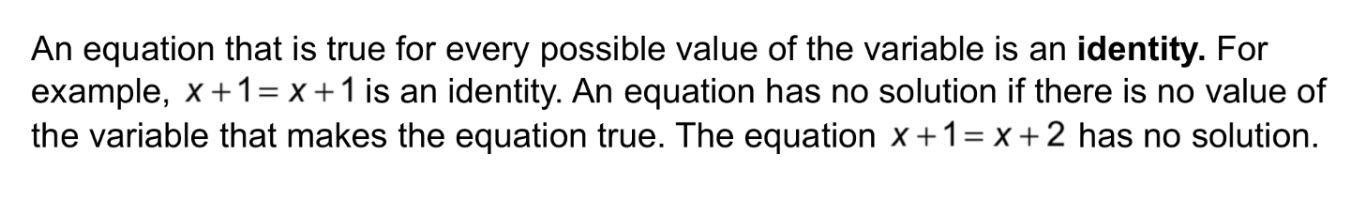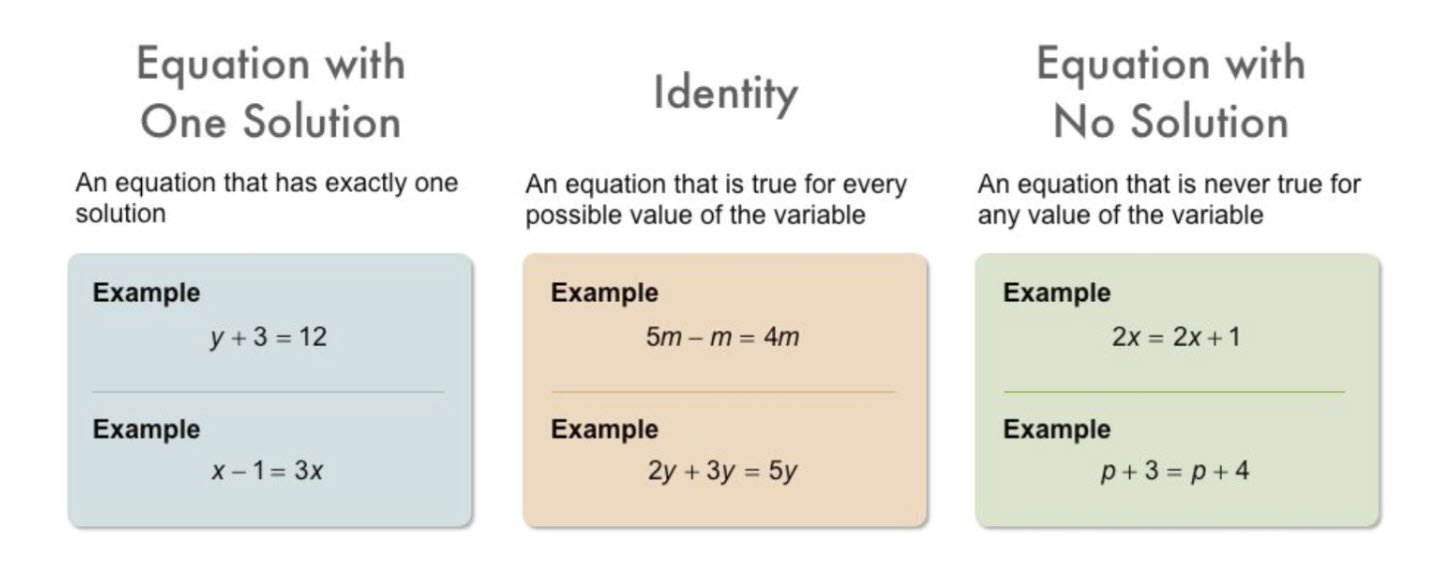Algebra 1 2-4 Guided Practice: Solving Equations with Variables on Both Sides
starstarstarstarstarstarstarstarstarstar
by Matthew Richardson
| 17 Questions1
2
1
10
Solve It! The diagram gives information about the populations of two towns. After how many years will the populations be equal?
Enter only a number.
2
10
Solve It! Explain how you found your response to the previous item.3
3
10
Take Note: Correctly order the steps below to describe the process (so far) for solving an equation with variables on both sides of the equation.
1. Combine like terms and use inverse operations to isolate the variable.
2. Check your solution by substituting it into the original equation.
3. Work to get the variables together on the same side of the equation and the constants on the other.
4
10
Problem 1 Got It? What is the solution of the equation?
Show your work on the canvas.
You may also complete your work on paper or on a whiteboard and upload a clear picture of it to the canvas.
-4
3
-6
125
5
10
Problem 1 Got It? Solve the equation from problem 1 by first subtracting 5x from each side instead of 2x. Do you get the same solution?
No, subtracting 5x yields a different solution.
Yes, 22 is the solution either way.6
6
10
Problem 2 Got It? An office manager spent \$650 on a new energy-saving copier that will reduce the monthly electric bill for the office from \$112 to \$88. In how many months will the copier pay for itself?
A
B
C
D7
7
10
Problem 3 Got It?
A
B
C
D8
8
10
Problem 3 Got It?
A
B
C
D9
10
11
12
13
9
10
Take Note: Describe what makes and equation an identity.
10
5
Take Note: Provide an example equation that has only one solution.
11
5
Take Note: Provide an example equation that has no solution.
12
5
Take Note: Provide an example equation that is an identity.
13
5
Take Note: How many solutions do identities have?
infinitely-many
zero
one
three
two14
14
10
Problem 4 Got It?
A
B
C
D15
15
10
Problem 4 Got It?
A
B
C
D16
10

Take Note: Place the five steps for solving equations in chronological order.
1. Combine like terms on each side of the equation.
2. Use the properties of equality to solve for the variable.
3. Check your solution in the original equation.
4. Use the properties of equality to get the variable terms on one side of the equation and the constants on the other.
5. Use the Distributive Property to remove any grouping symbols. Use properties of equality to clear decimals and fractions.17
17
10
Take Note: Summarize the mathematical content of this lesson. What topics, ideas, and vocabulary were introduced?